• 问题描述：matlab 条件判断语句不生效想用cos(w*t+y)的值的正负来决定函数的表达式,可是发现条件判断语句失效了,直接按最后一个条件语句运行了,w是角速度,y是初相位,均已赋值,t取0:0.004:1.主要函数部分：if cos(w*t...
问题描述：matlab 条件判断语句不生效想用cos(w*t+y)的值的正负来决定函数的表达式,可是发现条件判断语句失效了,直接按最后一个条件语句运行了,w是角速度,y是初相位,均已赋值,t取0:0.004:1.主要函数部分：if cos(w*t+y)1个回答分类：综合2014-09-22问题解答：我来补答上面的if-end结构用一下语句代替即可>> s=(atan((r*sin(w.*t+y)+d-r*sin(b01)*sin(a)*sin(a))./(r*cos(w.*t+y)))+pi).*(t<0)+(atan((r*sin(w.*t+y)+d-r*sin(b01)*sin(a)*sin(a))./(r*cos(w.*t+y)))).*(t>=0);有疑问,欢迎交流；如有用,及时采纳.再问： 我想要的是根据cos(w.*t+y)的值来判断，为什么你的语句中用的是t=0呢？再答： 打错，不好意思>> s=(atan((r*sin(w.*t+y)+d-r*sin(b01)*sin(a)*sin(a))./(r*cos(w.*t+y)))+pi).*(cos(w*t+y)<0)+(atan((r*sin(w.*t+y)+d-r*sin(b01)*sin(a)*sin(a))./(r*cos(w.*t+y)))).*(cos(w*t+y)>=0);这样没问题了。你的w如果不是标量，要加点>> s=(atan((r*sin(w.*t+y)+d-r*sin(b01)*sin(a)*sin(a))./(r*cos(w.*t+y)))+pi).*(cos(w.*t+y)<0)+(atan((r*sin(w.*t+y)+d-r*sin(b01)*sin(a)*sin(a))./(r*cos(w.*t+y)))).*(cos(w.*t+y)>=0); 还有疑问吗？我过会要下线了，如果有的话尽快说吧再问： 不好意思刚才洗了个澡 明天白天调试程序，如果没问题我就采纳你的了，非常感谢太棒了运行成功了，可以的话可以加个qq之类的联系么，我是matlab初学者希望以后可以多请教一些matlab的问题.........再答： 我不常用QQ的，多在知道交流交流，互相学习展开全文阅读
展开全文• MATLAB if 语句语法 在MATLAB中 的 if 语句的语法是： if <expression>% statement(s) will execute if the boolean expression is true <statements>end 表达式的计算结果如果是“true”，那么在...

一个 if 语句和一个布尔表达式后跟一个或多个语句，由 end 语句分隔，就是一个 if ... end 语句

MATLAB if 语句语法

在MATLAB中 的 if 语句的语法是：

if <expression>% statement(s) will execute if the boolean expression is true <statements>end

表达式的计算结果如果是“true”，那么在代码块中，如果语句会被执行。如果表达式计算结果为“false”，那么第一套代码结束后的语句会被执行。

MATLAB if 语句流程图：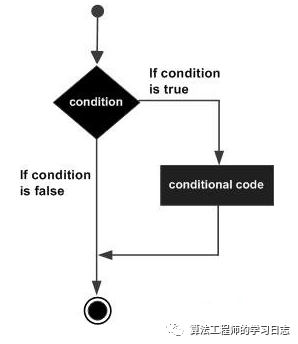详细例子如下：

在MATLAB中建立一个脚本文件，并输入下述代码：​​​​​​​

a = 10;% check the condition using if statement if a < 20 % if condition is true then print the following   fprintf('a is less than 20' );endfprintf('value of a is : %d', a);

运行该文件，显示下述结果：

​​​​​​​

a is less than 20value of a is : 10

在MATLAB的 if...else...end 语句中，if 语句后面可以跟一个可选择的 else 语句，当执行的表达式为假的时候，执行 else 语句。

if...else...end 语句语法：

MATLAB 中一个 if ... else 语句的语法示例：​​​​​​​

if <expression>% statement(s) will execute if the boolean expression is true <statement(s)>else<statement(s)>% statement(s) will execute if the boolean expression is false end

如果布尔表达式的值为 “true”，那么执行 if 的代码块；如果布尔表达式的值为 “false”，else 的代码块将被执行。

if...else...end 语句流程图：

详细例子如下：

在MATLAB中建立一个脚本文件，并输入下述的代码：​​​​​​​

a = 100;% check the boolean condition    if a < 20         % if condition is true then print the following        fprintf('a is less than 20' );   else       % if condition is false then print the following        fprintf('a is not less than 20' );   end   fprintf('value of a is : %d', a);

编译和执行上述代码，产生下述结果：​​​​​​​

a is not less than 20value of a is : 100

if...elseif...elseif...else...end 语句语法：

MATLAB 的 if...elseif...elseif...else...end 语句中 if 语句可以跟随一个（或多个）可选的 elseif... else 语句，这是非常有用的，可以用来对各种条件进行测试。

使用 if... elseif...elseif...else 语句，要注意以下几点：

一个 if 可以有零个或多个 else，但是它必须跟在 elseif 后面（即只有 elseif 存在才会有 else）。

一个 if 可以有零个或多个 elseif ，必须出现else。

一旦 elseif 匹配成功，余下的 elseif 将不会被测试。

if... elseif...else...end 语法：​​​​​​​

if <expression 1>% Executes when the expression 1 is true <statement(s)>elseif <expression 2>% Executes when the boolean expression 2 is true<statement(s)>Elseif <expression 3>% Executes when the boolean expression 3 is true <statement(s)>else %  executes when the none of the above condition is true <statement(s)>end

详细例子如下：

在MATLAB中建立一个脚本文件，并输入下述代码：​​​​​​​

a = 100;%check the boolean condition    if a == 10          % if condition is true then print the following        fprintf('Value of a is 10' );    elseif( a == 20 )       % if else if condition is true        fprintf('Value of a is 20' );    elseif a == 30         % if else if condition is true         fprintf('Value of a is 30' );   else        % if none of the conditions is true '       fprintf('None of the values are matching');   endfprintf('Exact value of a is: %d', a );

编译和执行上述代码，产生如下结果：​​​​​​​

None of the values are matchingExact value of a is: 100

展开全文if elseif else
• MATLAB中我们常常用到条件判断语句结构，通过实例介绍这个结构的用法： 1、if..end结构，运行下面的句子，此条件语句是判断5是否大于3，如果大于3，就将1赋值给； 2、if.elseend 结构，我们以如下内容进行判断： ...
MATLAB中我们常常用到条件判断语句结构，通过实例介绍这个结构的用法： 1、if..end结构，运行下面的句子，此条件语句是判断5是否大于3，如果大于3，就将1赋值给； 2、if.elseend 结构，我们以如下内容进行判断： 运行以上语句，结果如MATLAB中我们常常用到条件判断语句结构，下面就通过实例介绍一下这个结构的用法方法运行上面的句子，此条件语句是判断5是否大于3，如果大于3，就将1赋值给a嵌套if语句的语法如下： if % Executes when the boolean expression 1 is true if % Executes when the boolean expression 2 is true end end 例如： 创建脚本文件并在其中键入以下代码 ： a = 100; b = 200; % check the boolean condition ia1=1,a2=1，判断a1是否大于a2，显然不大于，然后就执行else语句，给a赋值为2,语法 if expression statements end MATLAB计算表达式，如果产生一个逻辑真或者非零结果，然后就执行一条或者多条MATLAB命令语句。 当有嵌套if时，每一个if必须和一个相应的end匹配。当你在if语句里面嵌套使用else if或者else时，一般的格式如下a1=a2=1,判断a1>a2?不满足，执行elseif语句，a1a&b或函数and(a,b)表示a和b做逻辑与运算，当a b全为非零时，运算结构为1，否则为0. if语句后的条件判断，两种方式均可以。扩展阅读，以下内容您可能还感兴趣。matlab if else语句怎么用？if     h(i,j)==a1(i,j)e(i,j)=a(i,j);elseif  h(i,j)==b1(i,j)e(i,j)=b(i,j);elseif h(i,j)==c1(i,j)e(i,j)=c(i,j);else   h(i,j)==d1(i,j)e(i,j)=d(i,j);end对这一段程序仔细分析，我没有仔细分析前面的程序，但可以猜测以下，h(i,j)肯定会和a1(i,j)、b1(i,j)、c1(i,j)、d1(i,j)中的某一个相等，这样当h(i,j)和a1(i,j)、b1(i,j)、c1(i,j)不相等的时候，就会执行到最后一条else语句else   h(i,j)==d1(i,j)e(i,j)=d(i,j);这时候ans=1；就会出现了。也就是Matlab把h(i,j)==d1(i,j)当做是一条单独的语句，而不是判断条件。究其原因，就是，if-elseif-else-end最后一个else是不用判断条件的，前面的条件都不满足就会执行else后的语句。再加上，用来计算的矩阵是由rand随机生成的，这样，就可以很好的解释为什么有时多，有时少，数量不确定的现象了。所以修改的办法是:1,把h(i,j)==d1(i,j)删除；2，把else改成elseif。对于这个程序，我感觉用if语句还是比较合理的，用switch-case可以用，但是它不适合这样的分支条件matlab中if语句如何嵌套使用？找出第一行是3，第二行是7,把这一行的第1个数换成10.clear allclca=[2 3 4 5 6;7 7 7 8 8]';for i=1:length(a)if(a(i,2)==7)if (a(i,1)==3)b(i,1)=10;elseb(i,1)=a(i,1);endb(i,2)=7;endend复制代码matlab中if语句实例，哪里出错了？x1是向量，一般不宜直接用于条件判断语句，例如，【x1<47】为真的条件是，x1所有的元素都小于47，类似这样的条件通常是不满足的，可能导致所有的if和elseif都不满足，最后只能执行else分支。考虑使用循环逐个元素进行判断。matlab if语句function dh=jiangyu (t,h)%降雨量的表达式if t<=400a=6*12*(0.122*10^(-3)-abs(0.00061*t*10^(-3)-0.122*10^(-3)))*cos(pi/9);elsea=0;end%液面高度微分方程中的其他部分：b=0.7*pi*(0.05)^2*sqrt(2*9.81*h);c=2*12*sqrt(0.15*h-h^2);%使结果符合客观事实,增加下面的判定z=isreal((a-b)/c);%如果液面高度正常为大于零的数，便按所设微分方程求解if z==1dh=(a-b)/c;%如果液面高度小于零，且此时不再下雨，则液面不再变化elseif (z==0 & a==0)dh=0;%如果液面高度小于零，但仍然下雨，则液面将会上升，速度为下式elseh=0.0001;dh=(a-b)/c;endend你的判断等于要改成 ==没有and这个用法的，用&表并列直接回车就行了你的c可能是虚数，注意~matlab怎么在if语句中再进行if语句？理论上可以嵌套的if语句个数不限，但是每一个if语句完成后一定要添加end语句与之对应，形式上如下所示：if 条件内容end或if 条件内容else if内容end或者if (expression1){commands1}else if (expression2){commands2}else if (expression3){commands3}else if ………………………………………else{commands}endendend……end
展开全文• 1 makefile中支持条件判断语句 可以根据条件的值来决定make的执行 可以比较两个不同变量或者变量和常量值注意事项 条件判断语句只能控制make实际执行的语句，但是不能控制规则中命令的执行过程 2 条件判断语句的...
1 makefile中支持条件判断语句

可以根据条件的值来决定make的执行
可以比较两个不同变量或者变量和常量值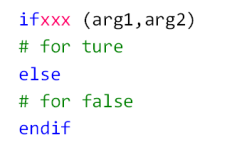注意事项 条件判断语句只能控制make实际执行的语句，但是不能控制规则中命令的执行过程
2 条件判断语句的语法说明

常用形式

ifxxx (arg1,arg2)
其它合法形式

ifxxx “arg1” “arg2”
ifxxx ‘arg1’ ‘arg2’
ifxxx ‘arg1’ “arg2”
ifxxx “arg1” ‘arg2’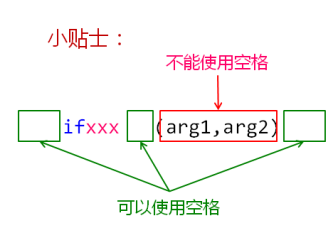条件判断关键字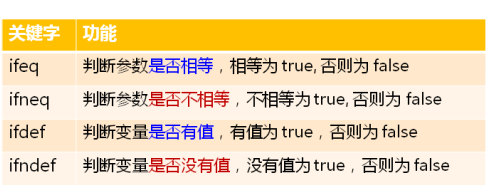编程实验：条件判断语句初探

.PHONY : test

var1 := A
var2 := $(var1) var3 := test: ifeq ($(var1),$(var2)) @echo "var1 == var2" else @echo "var1 != var2" endif ifneq ($(var2),)
@echo "var2 is NOT empty"
else
@echo "var2 is empty"
endif

ifdef var2
@echo "var2 is NOT empty"
else
@echo "var2 is empty"
endif

ifndef var3
@echo "var3 is empty"
else
@echo "var3 is NOT empty"
endif
输出结果：
var1 == var2
var2 is NOT empty
var2 is NOT empty
var3 is empty

3 一些工程经验

条件判断语句之前可以有空格，但不能Tab字符（’\t’）
在条件语句中不要使用自动变量（$@，$^， $<） 一条完整的语句必须位于同一个makefile中 条件判断类似C语言的宏，预处理阶段有效，执行阶段无效 make在加载makefile时 首先计算表达式的值（赋值方式不同，计算方式不同） 根据判断语句的表达式决定执行的内容 下面的代码执行结果相同吗？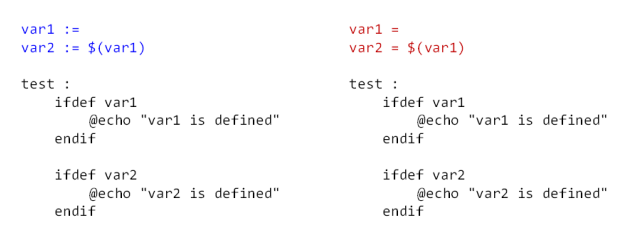编程实验：make行为 .PHONY : test var1 := var2 :=$(var1)

var3 =
var4 = \$(var3)

#var3 = 3

test:
ifdef var1
@echo "var1 is defined"
else
@echo "var1 is NOT defined"
endif

ifdef var2
@echo "var2 is defined"
else
@echo "var2 is NOT defined"
endif

ifdef var3
@echo "var3 is defined"
else
@echo "var3 is NOT defined"
endif

ifdef var4
@echo "var4 is defined"
else
@echo "var4 is NOT defined"
endif

输出结果：
var1 is NOT defined
var2 is NOT defined
var3 is NOT defined
var4 is defined

由于条件语句是在make加载makefile时进行处理的，所以当变量进行递
归赋值的时候，处理到条件判断语句时，无法确定在之后的语句中是否
改变了变量的值，所以make认为此变量非空。在make完成对makefile的
预处理后，所有变量的值都是确定的。但是，在本例中如果判断var3和
var4的值是否相等，却有一个与之矛盾的结果，输出结果为相同。按理
说一个非空，一个空应该不相等才是？

4 小结

条件判断根据条件的值来决定make的执行
条件判断可以比较两个不同的变量或者变量和常量值
条件判断在预处理阶段有效，在执行阶段无效
条件判断不能控制规则中命令的执行过程
修改记录

时间
动作
2017.7.16
首次上传
参考资料

唐老师 — 狄泰软件学院 — 十二月提升计划
展开全文• 文章导读【在生活中，常常会遇到许多需要判断的情况，在这种情况下，需要根据一些条件作出决定和选择。例如，在我们打算出门时，需要判断天气怎么样，如果下雨了，就要带上雨伞；外出旅行时，需要根据不同情况，选择...
• matlab中循环语句与for循环篇一：matlab循环语句循环结构1．for语句for语句的格式为：for 循环变量=表达式1:表达式2:表达式3循环体语句end其中表达式1的值为循环变量的初值，表达式2的值为步长，表达式3的值为循环...
• 环境：matlab 2018a 问题： if(3&lt;temp&lt;6) ... end 解决： (3&lt;temp&lt;6)写法不对，应改为： if(temp &lt; 3) &amp;&amp; (temp &lt; 6) 不知为神马
• matlab和octave都差不多(对于像我一样比较菜鸟的) 如题，代码如下：
• MATLAB条件分支语句： 1）if...end 2）if...else...end 3）if...elseif...elseif...else...end a=30; if a<20 fprintf('\na is less than 20\n'); else fprintf('a is larger than 20') end ...
• if (elseif…) else end 条件语句 循环语句： 高斯算法把帕斯卡矩阵转化为上三角形式： A=pascal(8) for j=1:7 for i=j+1:8 A(i,:)=A(i,:)-A(i,j)/A(j,j)*A(j,:) end end 迭代法求根： A1=[0 3/7 -2/...
• 条件判断语句 if 和c语言类似，直接看代码： if–else if-elseif-else: 判断三边是否可以组成三角形： ———————————————————————————————— ...
• matlab中for循环嵌套if语句1、新建一个子程序loopcolor。2、然后是定义几个变量，都是整数型。Dim myrow As Integer Dim mycol As Integer Dim mycolor As Integer。3、为mycolor赋值。mycolor = 0。4、对myrow进行...
• 经过前几篇的学习，我想对于Matlab的两种M文件都已经清楚各自的功能并对函数的相关知识有了一定的了解，...~Show Time~1条件控制语句在编写程序时，会根据需要，使用不同的条件控制语句，可以是判断语句也可以是分支...
• ## matlab中的if语句

万次阅读 2014-04-13 02:12:14
随时随地阅读更多技术实战干货，获取项目源码、学习资料，请关注源代码社区公众号(ydmsq666)、博主微信（guyun...MATLAB计算表达式，如果产生一个逻辑真或者非零结果，然后就执行一条或者多条MATLAB命令语句。 当有...matrix if statements
• while (max(abs([DeltaPi]))>pr && fr(1)+fr(2)+fr(3)>0.5 && fr(2)+fr(4)>0.5 && fr(3)+fr(5)>0.5 && fr(4)...想让以上五个条件同时满足，但是程序未进行循环，不满足任意条件，while语句可以实现满足多条件的功能吗？开发语言
• 1、3、4、 输出： var1 == var2 var2 is NOT empty var2 is NOT empty var3 is empty 5、6、7、 输出： var1 is NOT defined var2 is NOT defined var3 is NOT defined var4 is defined 8、
• MATLAB的基本语句结构直接赋值语句函数调用语句多样的函数调用机制冒号表达式子矩阵的提取等间距行向量的生成 MATLAB语句有两种最基本的结构——直接赋值结构和函数调用结构。 直接赋值语句 基本结构：赋值变量=...基础
• MATLAB中我们常常用到条件判断语句结构，通过实例介绍这个结构的用法：1、if.....end结构，运行下面的句子，此条件语句是判断5是否大于3，如果大于3，就将1赋值给；2、if....else...end 结构，我们以如下内容进行...
• 在编写MATLAB程序过程中，有时会遇到当程序运行到满足if条件时让程序跳出，停止运行的情况，在MATLAB中，使用return语句或者空语句实现程序跳出。如果使用return语句，在脚本文件会出现小问题，在函数文件中则会正常...
• 不管写什么程序，平时都会... switch这样的语句，来达到对条件判断。下面看来一段代码： function abc(test){ if (test == 1){ console.log('test的值是'+test); } else if (test == 2){ console.log('test
• 原文地址：matlab基本语句作者：我的视界matlab 基本语句 1.循环语句for fori=s1:s3：s2 循环语句组 end 解释：首先给i赋值s1；然后，判断i是否介于s1与s2之间；如果是，则执行循环语句组,i=i+s3（否则，退出循环....操作系统
• matlab第讲 条件语句和循环语句课堂》由会员分享，可在线阅读，更多相关《matlab第讲 条件语句和循环语句课堂(29页珍藏版)》请在人人文库网上搜索。1、Matlab,软件实习,1,第四讲,条件语句和循环语句,Matlab,软件...
• 条件语句可用于在运行时选择要执行的代码块。...对于if和switch，MATLAB 执行与第一个 true 条件相对应的代码，然后退出该代码块。每个条件语句都需要end关键字。 一般而言，如果具有多个可能的离散已知值，读取sw...
• 1、我们首先需要了解if语句是一种选择判断语句，可以和for语句结合使用，也可以单独使用，这里创建了3个变量，作出了一个简单判断如果a小于b，b等于a，需要注意的是if语句后面要有end语句结束。运行脚本之后，在...
• matlab中if语句判断两个变量是否相等，打印输出两个变量相等，但if语句就是不执行，why？ if语句判断两个变量是否相等出现问题 我出现的问题 if语句 if uwb_timestamp(uwb_iter)== SampleTimePoint(imu_iter) uwb_...
• 一、认识MATLAB1. MATLAB概述(1)在欧美各高等学校, Matlab成为线性代数、自动控制理论、数字信号处理、时间序列分析、动态系统仿真、图像处理等诸多课程的基本教学工具，成为本科生、硕士生和博士生的必须掌握的基本...
• switch 变量 case 判断值a 选择判断语句a case 判断值b 选择判断语句b …… otherwise 判断执行语句 end 举例： i=1; switch i case 1 i=11;break; c...switch case
• 功能：根据控制台输入的数字来判断它的大小 方法一、for循环实现 disp('数字比大小...') for i = 1:10 d=input('请输入一个数:') if(d<100) if(d<10) disp('这是一个小于10的数字') elseif(d <= ......

# matlab条件判断语句matlab 订阅